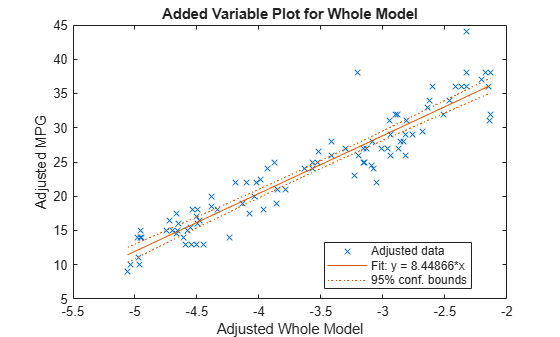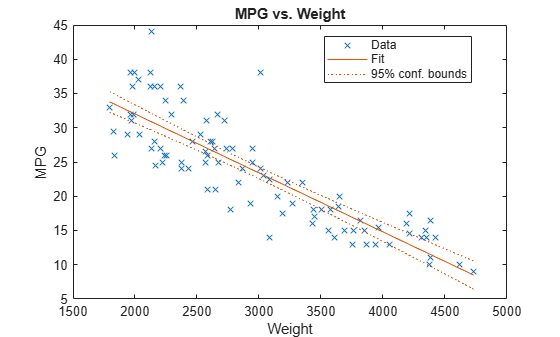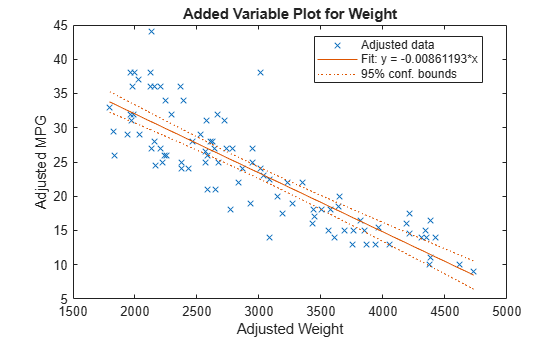# plot

Scatter plot or added variable plot of linear regression model

## Syntax

``plot(mdl)``
``plot(ax,mdl)``
``h = plot(___)``

## Description

example

````plot(mdl)` creates a plot of the linear regression model `mdl`. The plot type depends on the number of predictor variables. If `mdl` includes multiple predictor variables, `plot` creates an Added Variable Plot for the whole model except the constant (intercept) term, equivalent to `plotAdded(mdl)`.If `mdl` includes a single predictor variable, `plot` creates a scatter plot of the data along with a fitted curve and confidence bounds.If `mdl` does not include a predictor, `plot` creates a histogram of the residuals, equivalent to `plotResiduals(mdl)`. ```
````plot(ax,mdl)` creates the plot in the axes specified by `ax` instead of the current axes.```
````h = plot(___)` returns graphics objects for the lines or patch in the plot, using any of the input argument combinations in the previous syntaxes. Use `h` to modify the properties of a specific line or patch after you create the plot. For a list of properties, see Line Properties and Patch Properties.```

## Examples

collapse all

Create a linear regression model of car mileage as a function of weight and model year. Then create an added variable plot to see the significance of the model.

Create a linear regression model of mileage from the `carsmall` data set.

```load carsmall Year = categorical(Model_Year); tbl = table(MPG,Weight,Year); mdl = fitlm(tbl,'MPG ~ Year + Weight^2');```

Create an added variable plot of the model.

`plot(mdl)`The plot illustrates that the model is significant because a horizontal line does not fit between the confidence bounds.

Create the same plot by using the `plotAdded` function.

`plotAdded(mdl)`Create a scatter plot of data along with a fitted curve and confidence bounds for a simple linear regression model. A simple linear regression model includes only one predictor variable.

Create a simple linear regression model of mileage from the `carsmall` data set.

```load carsmall tbl = table(MPG,Weight); mdl = fitlm(tbl,'MPG ~ Weight')```
```mdl = Linear regression model: MPG ~ 1 + Weight Estimated Coefficients: Estimate SE tStat pValue __________ _________ _______ __________ (Intercept) 49.238 1.6411 30.002 2.7015e-49 Weight -0.0086119 0.0005348 -16.103 1.6434e-28 Number of observations: 94, Error degrees of freedom: 92 Root Mean Squared Error: 4.13 R-squared: 0.738, Adjusted R-Squared: 0.735 F-statistic vs. constant model: 259, p-value = 1.64e-28 ```

`pValue` of the `Weight` variable is very small, which means that the variable is statistically significant in the model. Visualize this result by creating a scatter plot of the data, along with a fitted curve and its 95% confidence bounds, using the `plot` function.

`plot(mdl)`The plot illustrates that the model is significant because a horizontal line does not fit between the confidence bounds, which is consistent with the `pValue` result.

Create the same plot by using the `plotAdded` function.

`plotAdded(mdl)`When a model includes only one term in addition to the constant term, an adjusted value is equivalent to its original value. Therefore, this added variable plot is the same as the scatter plot created by the `plot` function.

## Input Arguments

collapse all

Linear regression model, specified as a `LinearModel` object created using `fitlm` or `stepwiselm`.

Target axes, specified as an `Axes` object.

If you do not specify the axes and the current axes are Cartesian, then `plot` uses the current axes (`gca`). For more information on creating an `Axes` object, see `axes` and `gca`.

## Output Arguments

collapse all

Graphics objects corresponding to the lines or patch in the plot, returned as a graphics array. Use dot notation to query and set properties of graphics objects. For details, see Line Properties and Patch Properties.

If `mdl` includes one or more predictors, then `h(1)`, `h(2)`, `h(3)`, and `h(4)` correspond to adjusted data points, the fitted line, and the lower and upper bounds of the fitted line, respectively.

If `mdl` does not include a predictor, then `h` corresponds to the histogram of residuals.

collapse all

An added variable plot, also known as a partial regression leverage plot, illustrates the incremental effect on the response of specified terms caused by removing the effects of all other terms.

An added variable plot created by `plotAdded` with a single selected term corresponding to a single predictor variable includes these plots:

• Scatter plot of adjusted response values against adjusted predictor variable values

• Fitted line for adjusted response values as a function of adjusted predictor variable values

• 95% confidence bounds of the fitted line

The adjusted values are equal to the average of the variable plus the residuals of the variable fit to all predictors except the selected predictor. For example, consider an added variable plot for the first predictor variable x1. Fit the response variable y and the selected predictor variable x1 to all predictors except x1 as follows:

yi = gy(x2i, x3i, …, xpi) + ryi,

x1i = gx(x2i, x3i, …, xpi) + rxi,

where gy and gx are the fit of y and x1, respectively, against all predictors except the selected predictor (x1). ry and rx are the corresponding residual vectors. The subscript i represents the observation number. The adjusted value is the sum of the average value and the residual for each observation.

`$\begin{array}{c}{\stackrel{˜}{y}}_{i}=\overline{y}+{r}_{yi},\\ {\stackrel{˜}{x}}_{1i}={\overline{x}}_{1}+{r}_{xi},\end{array}$`

where ${\overline{x}}_{1}$ and $\overline{y}$ represent the average of x1 and y, respectively.

`plotAdded` plots a scatter plot of (${\stackrel{˜}{x}}_{1i}$, ${\stackrel{˜}{y}}_{i}$), a fitted line for $\stackrel{˜}{y}$ as a function of ${\stackrel{˜}{x}}_{1}$ (that is, ${\beta }_{1}{\stackrel{˜}{x}}_{1}$), and the 95% confidence bounds of the fitted line. The coefficient β1 is the same as the coefficient estimate of x1 in the full model, which includes all predictors.

ryi represents the part of the response values unexplained by the predictors (except x1), and rxi represents the part of the x1 values unexplained by the other predictors. Therefore, the fitted line represents how the new information introduced by adding x1 can explain the unexplained part of the response values. If the slope of the fitted line is close to zero and the confidence bounds can include a horizontal line, then the plot indicates that the new information from x1 does not explain the unexplained part of the response values well. That is, x1 is not significant in the model fit.

`plotAdded` also supports an extension of the added variable plot so that you can select multiple terms instead of a single term. Therefore, you can also specify a categorical predictor, all terms that involve a specific predictor, or the model as a whole (except a constant (intercept) term). Consider a set of predictors X with a coefficient vector β, where βi is the coefficient estimate of xi in the full model if you specify the ith coefficient for an added variable plot; otherwise, βi is zero. Define a unit direction vector u as u = β/s where s = norm(β). Then, Xβ = (Xu)s. Treat Xu as a single predictor with a coefficient s, and create an added variable plot for Xu in the same way as creating the plot for a single term. The coefficient of the fitted line in the added variable plot corresponds to s.

`plot` creates an added variable plot for the model as a whole (except a constant term ) if the model includes multiple terms.

## Tips

• The data cursor displays the values of the selected plot point in a data tip (small text box located next to the data point). The data tip includes the x-axis and y-axis values for the selected point, along with the observation name or number.